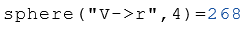# Class Sphere

Calculation of the properties of spheres

## Description

The class Sphere calculates properties of a sphere.

## Properties

The following properties can be queried as a result or passed as an argument.

#### Description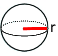r Radius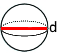d Diameter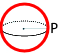P Perimeter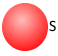S Surface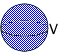V Volume

## Syntax

Object = Sphere ("arg names", arguments)

Value = Sphere ("get -> arg names", arguments)

## Example

Calculation of all properties from the radius $$r$$.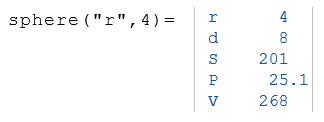The following example displays the volume $$V$$ from the object $$a$$.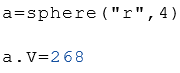Calculation of the volume $$V$$ with the radius $$r$$.